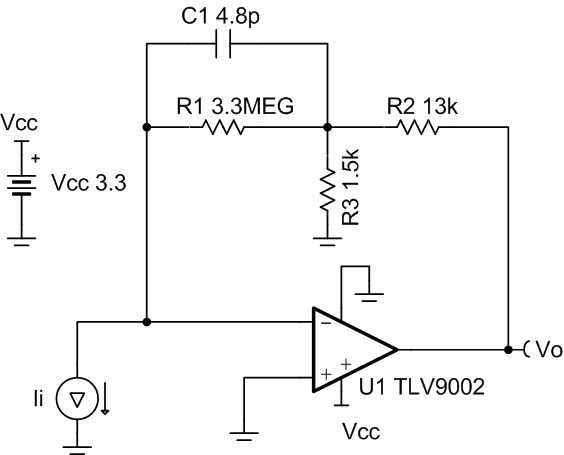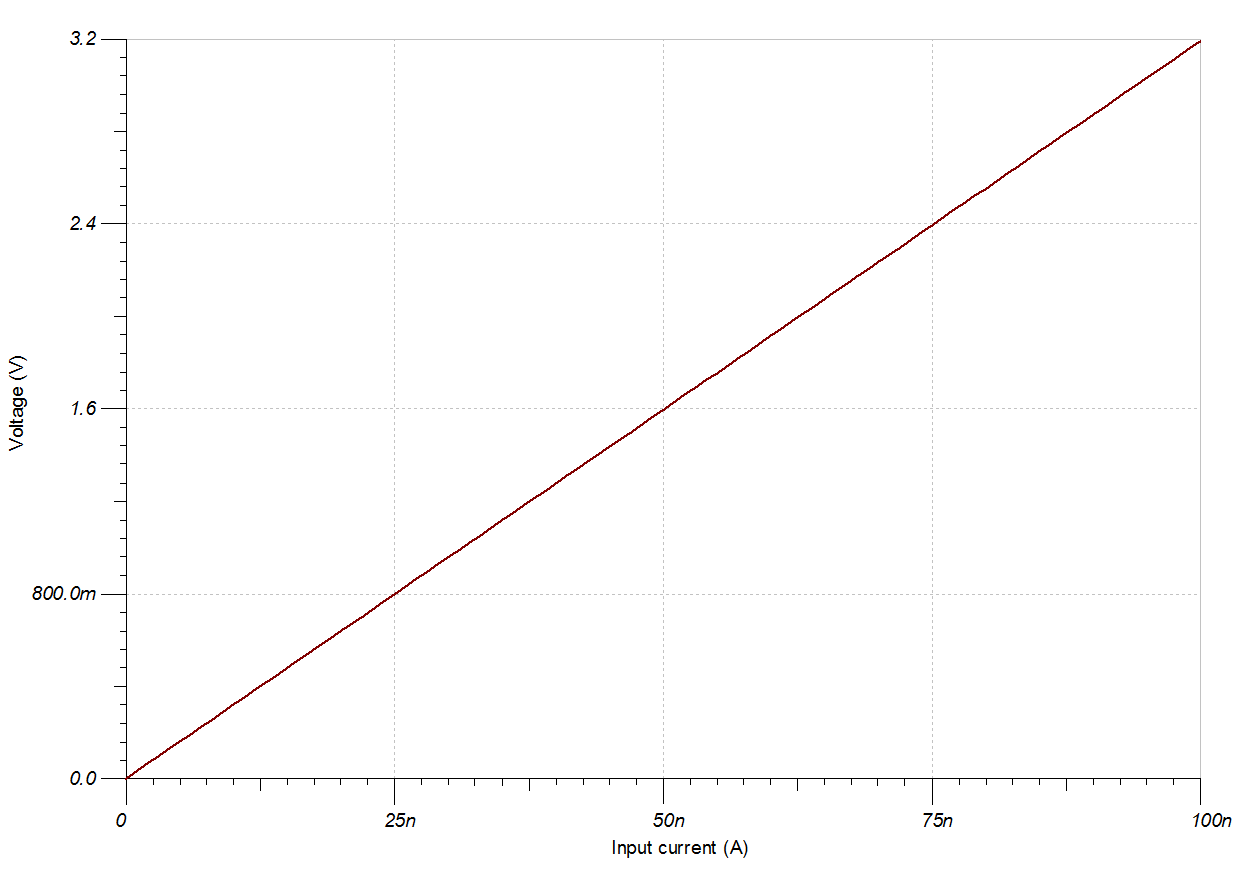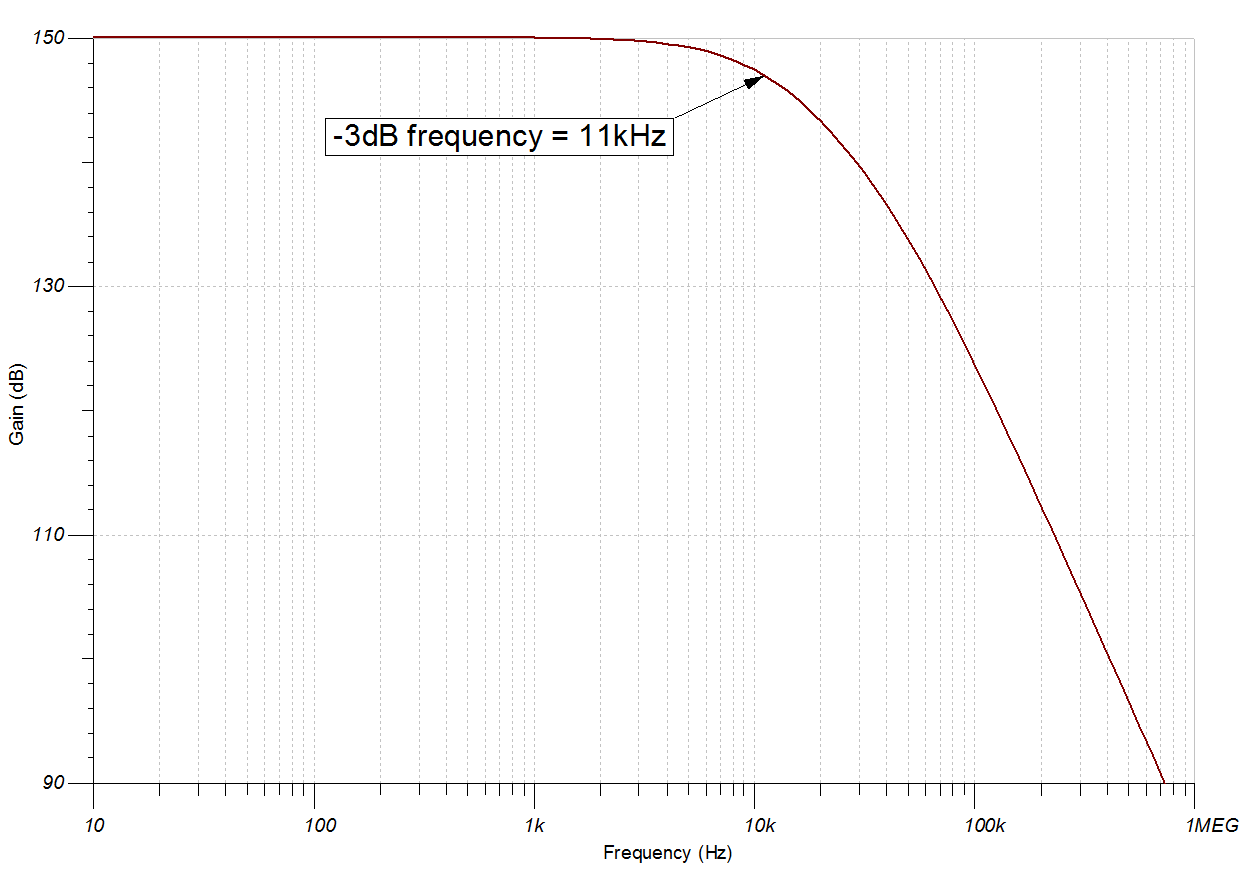SBOA284 March   2021

Design Goals

InputOutputBWSupply
IiMinIiMaxVoMinVoMaxfpVccVee
0A100nA0V3.2V10kHz3.3V0V

Design Description

This transimpedance amplifier with a T-network feedback configuration converts an input current into an output voltage. The current-to-voltage gain is based on the T-network equivalent resistance which is larger than any of the resistors used in the circuit. Therefore, the T-network feedback configuration circuit allows for very high gain without the use of large resistors in the feedback or a second gain stage, reducing noise, stability issues, and errors in the system.Design Notes

1. C1 and R1 set the input signal cutoff frequency, fp.
2. Capacitor C1 in parallel with R1 helps limit the bandwidth, reduce noise, and also improve the stability of the circuit if high-value resistors are used.
3. The common-mode voltage is the voltage at the non-inverting input and does not vary with input current.
4. A bias voltage can be added to the non-inverting input to bias the output voltage above the minimum output swing for 0A input current.
5. Using high-value resistors can degrade the phase margin of the circuit and introduce additional noise in the circuit.
6. Avoid placing capacitive loads directly on the output of the amplifier to minimize stability issues.
7. For more information on op amp linear operating region, stability, slew-induced distortion, capacitive load drive, driving ADCs, and bandwidth see the Design References section.

Design Steps

The transfer function of this circuit follows:

Equation 1.
1. Calculate the required gain:
Equation 1.
2. Choose the resistor values to set the pass-band gain:
Equation 1.

Since R1 will be the largest resistor value in the system choose this value first then choose R2 and calculate R3. Select R1 = 3.3MΩ and R2 = 13kΩ. R1 is very large due to the large transimpedance gain of the circuit. R2 is in the ~10k ohm range so the op amp can drive it easily.

Equation 1. ${R}_{3}=\left(\frac{{R}_{2}×{R}_{1}}{Gain–{R}_{1}–{R}_{2}}\right)=\left(\frac{\text{13kΩ × 3.3MΩ}}{{\text{3.2 × 10}}^{\text{7}}\frac{\text{V}}{\text{A}}\text{– 3.3MΩ – 13kΩ}}\right)\text{= 1.5kΩ}$
3. Calculate C1 to set the location of fp.
Equation 1.
4. Run a stability analysis to make sure that the circuit is stable. For more information on how to run a stability analysis see the TI Precision Labs - Op amp: Stability video.

Design Simulations

DC Simulation ResultsAC Simulation ResultsDesign References

1. See Analog Engineer's Circuit Cookbooks for TI's comprehensive circuit library.
2. See SPICE file, SBOMB39.
3. See TIPD176, www.ti.com/tool/tipd176.
4. For more information on many op amp topics including common-mode range, output swing, bandwidth, and how to drive an ADC please visit TI Precision Labs.

Design Featured Op Amp

TLV9002
Vcc1.8V to 5.5V
VinCMRail-to-rail
VoutRail–to–rail
Vos0.4mV
Iq60µA
Ib5pA
UGBW1MHz
SR2V/µs
#Channels1, 2, 4
www.ti.com/product/TLV9002

Design Alternate Op Amp

OPA375
Vcc 2.25V to 5.5V
VinCM Vee to (Vcc –1.2V)
VoutRail–to–rail
Vos0.15mV
Iq890µA
Ib10pA
UGBW10MHz
SR4.75V/µs
#Channels1
www.ti.com/product/OPA375